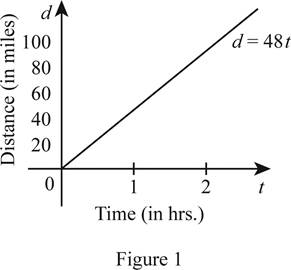# To express: The distance in terms of the time elapsed.### Single Variable Calculus: Concepts...

4th Edition
James Stewart
Publisher: Cengage Learning
ISBN: 9781337687805### Single Variable Calculus: Concepts...

4th Edition
James Stewart
Publisher: Cengage Learning
ISBN: 9781337687805

#### Solutions

Chapter 1.2, Problem 14E

(a)

To determine

## To express: The distance in terms of the time elapsed.

Expert Solution

The distance d in terms of time elapsed is, d=48t .

### Explanation of Solution

Let d be the distance traveled in miles and the t be the time elapsed in hours.

When t = 0, the distance is 0 which can be expressed as (0, 0).

Since he passes the Ann Arbor at 50 minutes, t = 50 minutes or t=56hrs.

The distance traveled is 40 miles. That is, at t=56hrs. , d=40 which can be expressed as (56,40) .

Use the obtained two points and find the slope m as follows.

m=400560=40×65=48

Thus, the slope m=48 .

Use the slope m=48 and the point (0, 0) and obtain the equation of distance d in terms of time elapsed t as follows.

yy1=m(tx1)d0=48(t0)d=48t

Thus, the required function is d=48t .

(b)

To determine

Expert Solution

### Explanation of Solution

Let x-axis be represented the time in hours and the y-axis be represented the distance in miles.

Given that the distance function of elapsed time is d=40t .

Obtain the value of d for various values of t and draw the graph of d as shown below in Figure 1.From Figure 1, it is observed that function is a linear function.

(c)

To determine

### To find: The slope of the graph drawn in part (b); explain the meaning of slope.

Expert Solution

The slope is 48, which represents the rate of change of the distance in miles.

### Explanation of Solution

The equation d=40t is in the form of y=mx+c in which m is slope and c is the y-intercept.

Thus, the slope of the Figure 1 shown in part (b) is 48. It represents that the rate of change of the distance in miles.

Moreover, it is observed that there is a decrease in the distance when time increases and hence it is in direct variation. The distance covered in an hour is 48 miles.

### Have a homework question?

Subscribe to bartleby learn! Ask subject matter experts 30 homework questions each month. Plus, you’ll have access to millions of step-by-step textbook answers!